Contour-Shifting with the IceCast Package

Abstract

This vignette illustrates how to apply Contour-Shifting to bias correct predictions with the IceCast R package. We will demonstrate how the functions in this package can be used to correct the bias in dynamical ensemble sea ice forecasts. If only bias correction (not model calibraion) is desired, many users will only need to use one function for simple bias correction: quick_run. This function takes in NetCDF files of observations and predictions and produces a netCDF file with bias-corrected predictions. This approach is outlined in the first section of the vignette. The remainder of the vignette focuses on how Contour-Shifting is executed and how the correction is determined. Users should be aware Contour-Shifting will only work well when there are a sufficient number of years of predictions and observations to build a reasonable statistical model.

In the vignette, we will illustrate Contour-Shifting using one example month. We will bias-correct the September 2008 ensemble prediction at a 2.5-month lead time using retrospective predictions and observations from 1993-2007. We will evaluate these post-processing techniques on model output from the 25-member ensemble from the European Centre for Medium-Range Weather Forecasts (ECMWF) available from the Copernicus Climate Change Service datastore and the Sea Ice Prediction Network Predictability Portal. We have converted the data to a Polar stereographic grid. We will also use observations of the monthly sea ice concentration obtained from the National Aeronautics and Space Administration (NASA) satellites Nimbus-7 SMMR and DMSP SSM/I-SSMIS and processed by the bootstrap algorithm. The data are distributed by the National Snow and Ice Data Center (NSIDC) (Comiso 2017). We have simplified the land mask of the observations to match the resolution from the model output.

Note: This vignette has been updated in IceCast Version 2 to incorporate updated data and methods used in Director et al (2019+). In particular, the lines on which the sea ice is mapped are now fixed for all regions, not just the Central Arctic Region. Additionally, the bootstrap observations have been updated to a newer version, predictions from the ensemble are now computed in the same way as the Sea Ice Outlook (Sea Ice Prediction Network, 2017), a larger number of regions are used, and the ECMWF ensemble is used. For details on how to exactly replicate Director et al. (2017), see the vignette in IceCast Version 1.1.

We will first load the IceCast package. We will also load the fields package (Nychka et al. 2016). While the fields package is not needed to use the functions in the IceCast package, it provides useful functions for generating spatial figures, which we will do in this vignette.

library("IceCast")
library("fields")

quick_run Function

We will first explain the quick_run function, which executes the full process of Contour-Shifting. It takes in two NetCDF files that contain the observed and predicted sea ice concentrations and outputs a NetCDF file that contains the bias-corrected sea ice concentration predictions. Users need to specify the file paths for the NetCDF file to be read in and where the new NetCDF file should be written. They also need to specify what month and year(s) they want to correct.

Prediction and observation data should be formatted as a NetCDF file with a single array with 4 dimensions (years x months x lon x lat). In the prediction array, each entry should have a value between 0 and 1 that indicates the sea ice concentration (as a proportion) or a value of NA that indicates land. The variable should be named ice_ind. The observation array defaults to having values that correspond to conventions of the NASA Bootstrap data where values betweeen 0 and 100 indicate the sea ice concentration percentage, values of 110 indicate the grid box is within the satellite hole, and values of 120 indicate the grid box is on land. The variable should be named conc. Alternatively, the observation values can be formatted the same as the prediction values. That is, each entry will have a value between 0 and 1 that indicates the sea ice concentration proportion or a value of NA that indicates land. For this case, select, dat_typ_obs = "simple" and name the variable ice_ind.

Below is an example of what a call to the quick_run function look likes. During its execution, a message will be printed as each year is mapped and each year is bias-corrected. You should expect about 1 minute of run-time for each year mapped and an additional 1 minute of run-time for each year bias corrected. The resulting NetCDF file of the bias-corrected prediction will be saved to the specified file at the functions’ completion. Note that the polygons created by Contour-Shifting are not constrained to exactly align with the grid. So, for exporting to a NetCDF file, each grid box is categorized as containing sea ice only if its center point is covered by sea ice.

##Not run##
quick_run(obs_NCDF = "/obs.nc", pred_NCDF = "/pred.nc", pred_years = 2008,
start_year = 1993, month = 2, output_file = "/outputFile.nc", level = 15,
dat_type_obs = "bootstrap")

We will now look at tools for data processing. The package has functions to easily read in binary observation data downloaded from NSIDC. To keep the package to a reasonable size, we have not uploaded binary files. To use these functions, the binary files must be named using the original file names used by NSIDC (i.e. bt_198301_n07_v02_n.bin). For example, we could load a raw binary files as follows

##Not run##

This gives a vector of numbers which encode information related to the concentration and land mask. We can convert this to a useful matrix using the read_monthly_BS function. To bias correct the September 2008 model output, we need to read in the observations from 1993-2007. This can be done with the read_monthly_BS command as follows

##Not run##
observed <- read_monthly_BS(start_year = 1993,end_year = 2007,
file_folder = "myFilePath/", version = 2)
obs_sept <- observed[, 9, , ] #Use September data only

where the folder “myFilePath/” is a path to a folder with all the NSIDC binary files. However, to keep the IceCast package to a reasonable size, we have not uploaded the binary files. Instead, the package includes some sample results from this function where the start_year is 2006 and the end_year is 2007. The results are stored as the obsSep2006_2007 array. This array, of dimension 2 x 12 x 304 x 448, gives the observed concentration fields for the years 2006-2007 for all twelve months.

Let’s look at the field for September 2007:

image.plot(obsSep2006_2007[length(2006:2007),,],
main = "Observed Sea Ice Concentration \n September 2007",
xaxt = "n", yaxt = "n")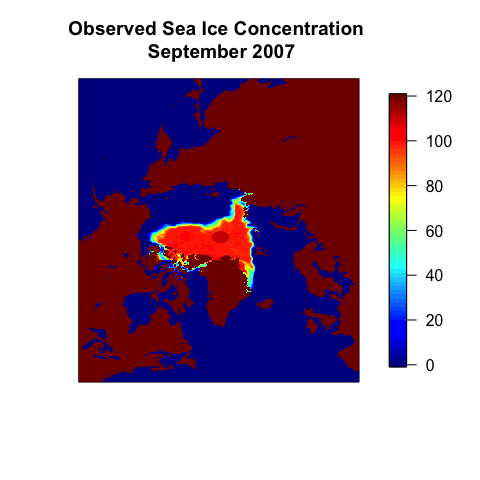Predictions

For making comparisons with observations, we need to specify an array of concentration values with dimensions year x month x longitude x latitude. The package doesn’t include built-in functions for loading predictions, since there are a wide range of dynamic ensemble models for sea ice on several different grids. For demonstration purposes, the IceCast package includes one set of prediction data taken as the sea ice probability, stored as the object sipSep2006_2007. This array has the ensemble predictions initalized in July from the ECMWF model as discussed in the introduction.

As an example, let’s look at the prediction for September 2008 at a 2.5-month lead time.

image.plot(sipSep2006_2007[2,,],
main = "Predicted Sea Ice Concentration \n September 2007 (2.5-month lead time)",
xaxt = "n", yaxt = "n")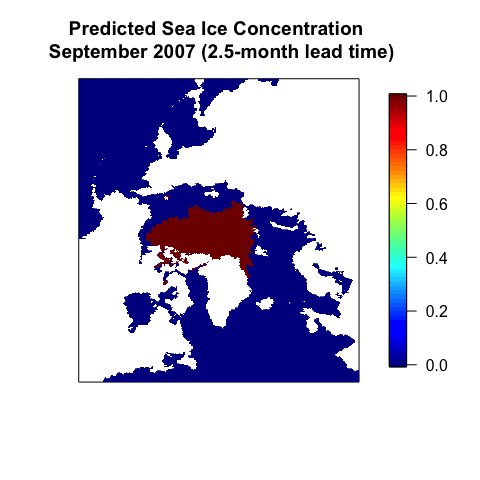Regions

We have several polygon objects built-in to IceCast for convenience. We have a polygon land that corresponds to the land. We can plot it as follows:

plot(land, col = "grey", main = "Land")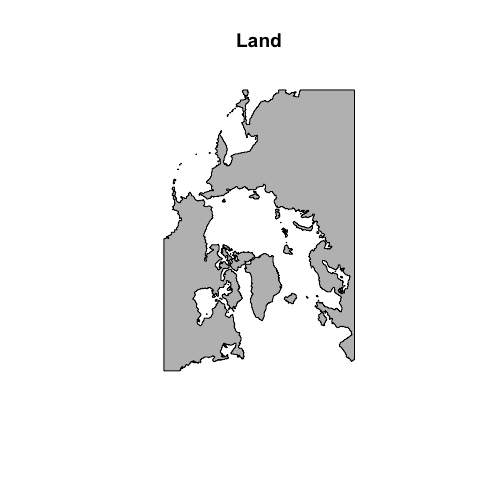The package also has several other built-in polygons. First, we have a polygon that gives all the combined regions that form the seas of the Arctic as defined by NSIDC. This polygon is named all_regions. We also have the polygon, bg_water, which gives all the regions on the polar stereographic grid that are not considered to be part of the seas of the Arctic. All the polygons are loaded automatically when the package is loaded. More details about these polygons can be found in their help pages. We’ll plot the regions now to visualize.

plot(land, col = "grey", main = "Seas of the Arctic")
plot(bg_water, col = "black", add = T)
plot(all_regions, col = "blue", add = T)
legend("bottom", fill = c("blue", "black"), cex = 0.75,
legend = c("Regions", "Outside Regions"))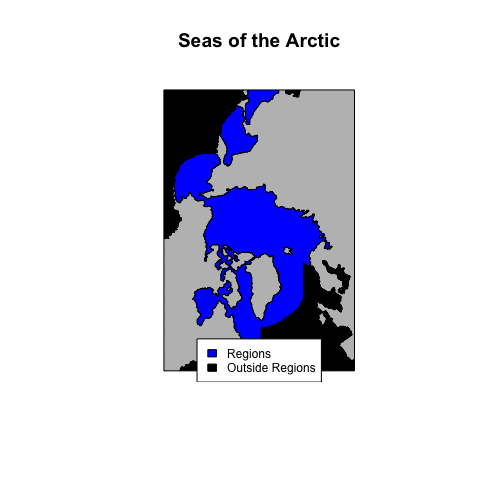Mapping ice sections

Built-in objects

For mapping the ice sections, we have some built-in regions and lines that are stored in the reg_info object (see the documentation on this object for more info). The package contains a reg_info object in the reg_info.rda file, which is typically what is used for all analysis. However, it would be possible to re-define the regions if desired by making a new reg_info object. We can use the reg_info object to plot the regions and the lines on which they are mapped.

colors <- c("darkblue", "green", "blue", "red", "orange", "yellow", "purple",
"pink",   "lightgreen", "brown", "tan", "darkgreen", "hotpink",
"navy", "beige", "darkblue", "green", "blue", "red", "orange",
"yellow")
plot(land, col = "grey", main = "Mapping Lines & Regions")
nReg <- length(reg_info$regions) for (i in 1:nReg) { plot(reg_info$regions[[i]], add = T, lwd = 1.5)
}
for (i in 2:nReg) {
plot(reg_info$start_lines[[i]], col = colors[i], add = T, lwd = 2) }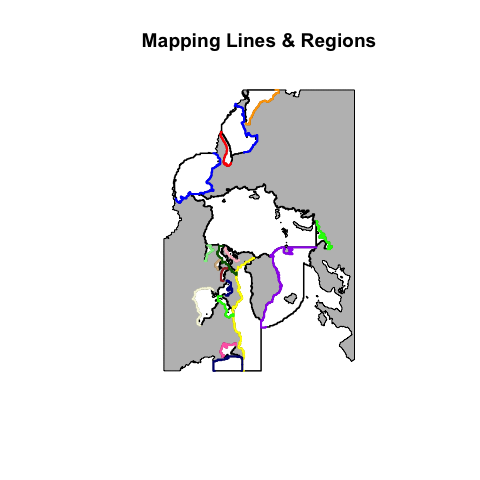Similarily, we can use the reg_info object to plot the regions and the lines that will intersect with the ice in the central Arctic region. #Find angle of mapping line (and color code) nLines <- length(reg_info$lines[])
ang <- rep(NA, nLines)
for (i in 1:nLines) {
temp <- reg_info$lines[][[i]]@lines[]@Lines[]@coords nTemp <- nrow(temp) ang[i] <- atan2(temp[nTemp, 2] - temp[1, 2], temp[nTemp, 1] - temp[1, 1]) } bp <- seq(-pi, pi, length.out = 65) angCol <- rainbow(length(ang)) #plot region and lines plot(reg_info$regions[], main = "Central Arctic Boundary Lines")
plot(land, add = T, col = "grey")
for (s in 1:length(reg_info$lines[])) { plot(reg_info$lines[][[s]], col = angCol[s], add = T)
}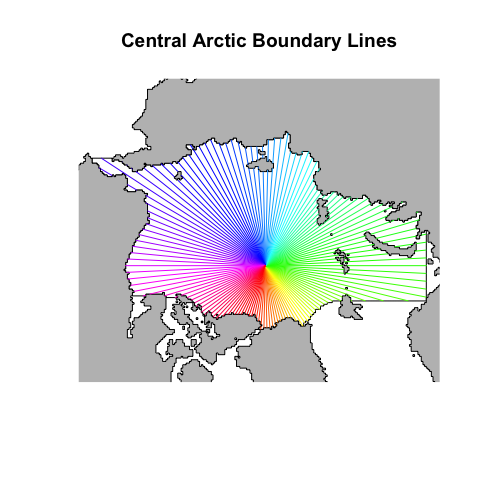Learn Mappings

With any prediction or observation, we can build a mapping. We will use the obsSep2006_2007 and sipSep2006_2007 arrays to demonstrate this, which are arrays with the observed data and model predictions for 2006-2007. For September 2007, we can map the region as follows

obs <- get_region(dat = obsSep2006_2007[length(2006:2007), ,],
dat_type = "bootstrap", level = 15)
obs_map <- get_map(ice = obs, plotting = TRUE, reg_info,
main = "Observed Mapping \n September 2007")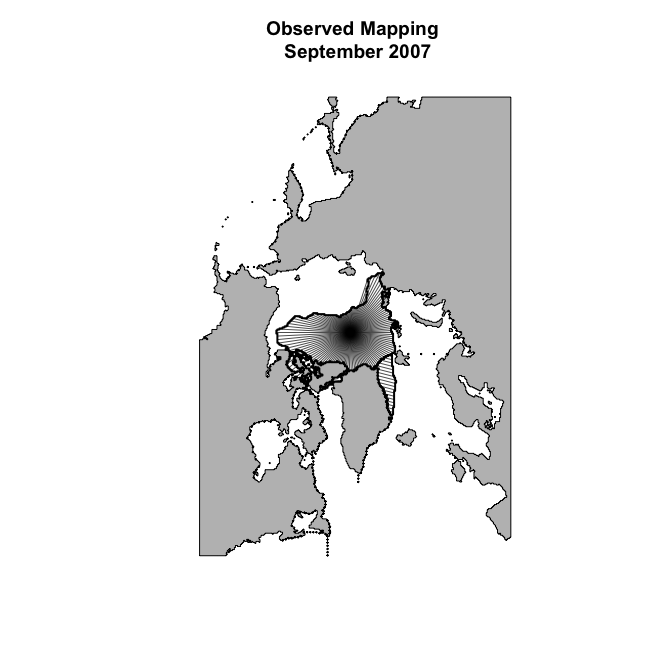To map and store results for a period of years, we use the create_mapping function. We will run this for a single year, with plotting turned on, as a demo.

par(mfrow = c(2, 2), oma = rep(0, 4), mar = c(1, 1, 2, 1))
discrep_demo1 <- create_mapping(start_year = 2007, end_year = 2007,
obs_start_year = 2006, pred_start_year = 2006,
observed = obsSep2006_2007, predicted = sipSep2006_2007[],
reg_info,  month = 9, level = 15,
dat_type_obs = "bootstrap", dat_type_pred = "simple",
plotting = TRUE)
##  "mapping complete for year 2007"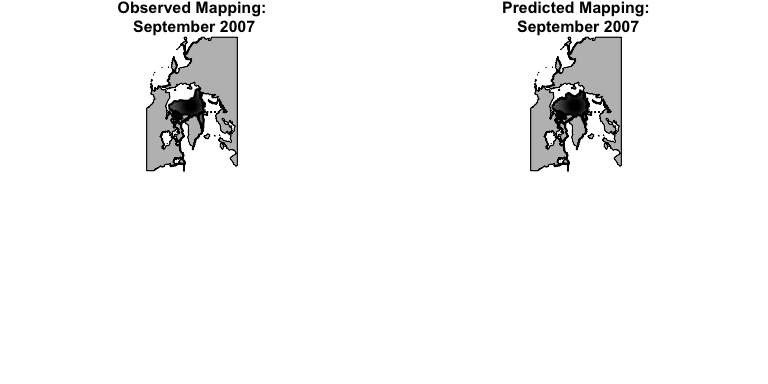The create_mapping function returns a list of four objects. The objects start_year and end_year give the first and last year that were mapped. The objects obs_list and pred_list are lists of arrays with one 3-dimensional array for each region. The first dimension is for the year. The other two dimensions form a matrix where each row corresponds to a point in the region’s fixed line. The seven columns give the fixed points’ x-coordinates, the fixed points’ y-coordinates, the mapped points’ x-coordinates, the mapped points’ y-coordinates, the length of the mapping vectors in the x-direction, the length of the mapping vectors in the y-direction, and the angle of the mapping vectors. Looking at the first few lines of the first region gives us an example of this.

##      [,1] [,2]      [,3]      [,4]      [,5]      [,6]
## [1,] -170  100 -475.0000 -600.1663 -305.0000 -700.1663
## [2,] -170  100 -444.9562 -625.0000 -274.9562 -725.0000
## [3,] -170  100 -413.6898 -650.0000 -243.6898 -750.0000
## [4,] -170  100 -374.2031 -650.0000 -204.2031 -750.0000
## [5,] -170  100 -330.2181 -625.0000 -160.2181 -725.0000
## [6,] -170  100 -300.0000 -661.1466 -130.0000 -761.1466

To bias correct September 2008, we will need the mappings for all the prior years (1993-2007). We could obtain them with something like the following command where observed and sip are arrays with the observed and ensemble sea ice probability data for all years up to 2007.

##Not run##
discrep <- create_mapping(start_year = 1993, end_year = 2007,
obs_start_year = 1993, pred_start_year = 1993,
observed = observed[,month,,],predicted = sip[,month,,],
reg_info, month,level = 15, dat_type_obs = "bootstrap",
dat_type_pred = "simple", plotting = TRUE)

However, since this command takes a bit of time to run, we’ve pre-loaded the results in the package as the object discrep.

Applying the bias correction

With the mappings for previous years completed, we’re now ready to bias correct the dynamic ensemble model prediction for September 2008. We can do this with just one line:

adj <- contour_shift(maps = discrep, predicted = sipSep2008, bc_year = 2008,
pred_start_year = 2008, reg_info,
level = NA, dat_type_pred = "simple")

The adjusted prediction, adj, is a SpatialPolygons object that gives the bias-corrected prediction of where we expect to see sea ice.

We can compare this polygon to the corresponding observation and unadjusted prediction. First we’ll convert the corresponding observation and prediction into polygons.

obs <- get_region(dat = obsSep2008, dat_type = 'bootstrap', level = 15)
un_adj <- get_region(dat = sipSep2008, dat_type = 'gfdl', level = 15)

We can now plot the results

plot(land, col = "grey", border = F,
main = "Predicted vs. Bias−Corrected Contours \n September 2008 (2.5-Month Lead Time)")
plot(obs, col = "lightblue", add = T, border = F)
plot(un_adj, border = "red", add = T)
plot(adj, border = "navy", add = T)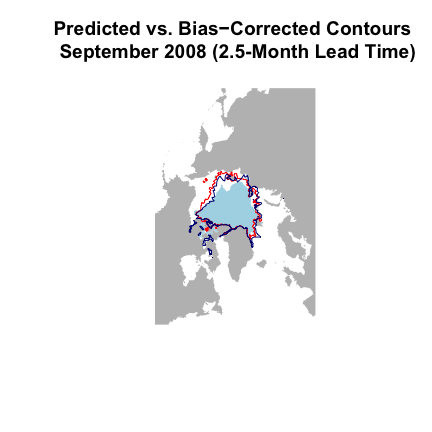We can see that the bias-corrected contour follows the observed region more closely than the unobserved prediction. We can also quantify by how much we have reduced the error. To do this, we first find the regions that are incorrectly predicted.

We’ll plot the overestimated regions in green and the underestimated regions in yellow.

par(mfrow = c(1, 2), oma = rep(0, 4), mar = rep(0, 4))
plot(land, col = "grey", border = FALSE, main = "Error Regions:\n Unadjusted")
plot(obs, col = "lightblue", border = F, add = T)
plot(over_est_un_adj, col = "green", border = F, add = T)
plot(under_est_un_adj, col = "yellow", border = F, add = T)
plot(un_adj, add = T, border = "red")

#bias-corrected
plot(land, col = "grey", border = FALSE, main = "Error Regions:\n Bias-corrected")
plot(obs, col = "lightblue", border = F, add = T)
plot(over_est_adj, col = "green", border = F, add = T)
plot(under_est_adj, col = "yellow", border = F, add = T)
plot(adj, add = T, border = "navy")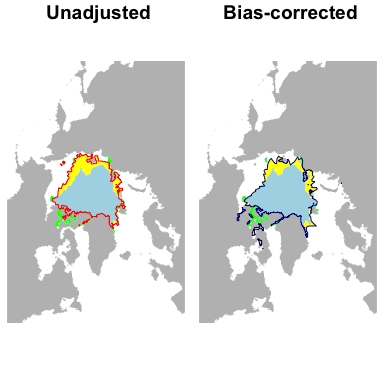If we calculate the areas of these regions and sum them up, we can find the total area in error, referred to as the Integrated Ice-Edge Error (IIEE) (Goessling et al. 2016). We can also find the difference between the IIEE for the unadjusted and bias-corrected results. By default, areas are reported in square kilometers, but we’ll report the result in to $$10^{5}$$ $$km^{2}$$. This gives